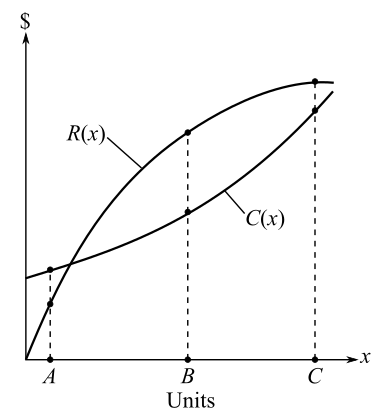Chapter 9, Problem 108RE### Mathematical Applications for the ...

11th Edition
Ronald J. Harshbarger + 1 other
ISBN: 9781305108042

#### Solutions

Chapter
Section### Mathematical Applications for the ...

11th Edition
Ronald J. Harshbarger + 1 other
ISBN: 9781305108042
Textbook Problem

# Cost, revenue, and profit The graph shows the total revenue and total cost functions for a company. Use the graph to decide (and justify) at which of points A, B, and C(a) the revenue from the next item will be least.(b) the profit will be greatest.(c) the profit from the sale of the next item will be greatest.(d) the next item sold will reduce the profit.(a)

To determine

The point on the graph, where the revenue from the next item will be the least,Explanation

Given Information:

The provided graph represents the total revenue and total cost functions for the company,

Explanation:

Consider the provided graph,

The point where the revenue from the next item will be the least can be found using the derivatives...

(b)

To determine

The point on the graph, where the profit from the next item will be the greatest,(c)

To determine

The point on the graph, where the profit from the next item will be the greatest,(d)

To determine

The point on the graph, where the next item sold reduces the profit,### Still sussing out bartleby?

Check out a sample textbook solution.

See a sample solution

#### The Solution to Your Study Problems

Bartleby provides explanations to thousands of textbook problems written by our experts, many with advanced degrees!

Get Started

#### Evaluate the indefinite integral. x1+x4dx

Single Variable Calculus: Early Transcendentals

#### j × (−k) = i −i j + k −j − k

Study Guide for Stewart's Multivariable Calculus, 8th

#### True or False: converges mean exists.

Study Guide for Stewart's Single Variable Calculus: Early Transcendentals, 8th